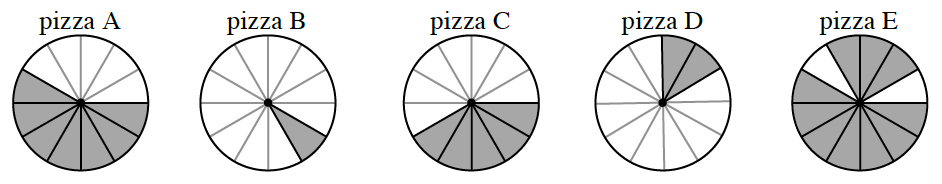Home > CC2MN > Chapter cc23 > Lesson cc23.1.1 > Problem3-10

3-10.

After a pizza party, Julia has parts of five pizzas left over, as shown below. Each pizza was originally cut into $12$ pieces, and the shaded areas represent the slices that were not eaten. Homework Help ✎1. What fraction of pizza A is left?

How many pieces are left (shaded)?

2. If all of the pieces were put together, how many whole pizzas could Julia make? How many extra pieces would she have?

Which parts can be put together to make a whole pizza?

Explain how there are $2$ whole pieces plus one extra piece left.

3. Julia wants to write the amount of leftover pizza as a single fraction. How could she do this?

How many slices are left?

$\frac{?}{12}$